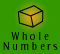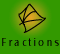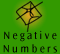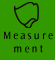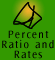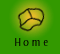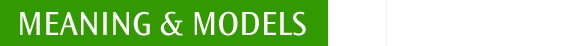| What do we mean by measurement? | Standard units of measure |
| Quick quiz |

What do we mean by measurement?

When we measure an object we quantify an attribute of the object such as,

the length of a pencil,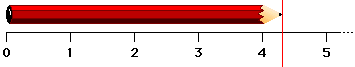the area of a yard, the capacity of a bottle,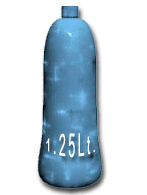or the mass of a brick,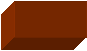A measurement always consists of a number and a unit. For example, that pencil is 4.3 cm long. The area of the yard is 100 m2.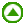Standard units of measure

SI Units

The International System of Units has defined a metric system of units to measure all physical quantities in the universe.

Three of the seven basic SI units are relevant to our everyday experiences. They are:

 quantity to measure SI unit abbreviation length metre m mass kilogram kg time second s

NOTE: These are standard abbreviations, other abbreviations which are strictly not correct are used occasionally. As teachers we need to make an effort to adhere to the standard abbreviations but accept other common forms.

Common prefixes for SI units

We can combine these basic units with prefixes to form a unit multiple of more convenient size. See the table below for some of these commonly used prefixes.

 prefix and symbol value and meaning example mega (M) 1 000 000 one million a megalitre is one million litres kilo (k) 1 000 one thousand a kilogram is one thousand grams deci (d) 0.1 one tenth a decimetre is one tenth of a metre centi (c) 0.01 one hundredth a centimetre is one hundredth of a metre milli (m) 0.001 one thousandth a milligram is one thousandth of a gram micro ( µ) 0.000 0001 one millionth a micrometre is one millionth of a metre. µ is a Greek letter, pronounced as 'mu'.

Because the metric system is based on 10 and its multiples we can easily alter the measurements to larger or smaller units. For example, to convert metres to centimetres we merely need to multiply by 100.

Primary school measurement topics include:

 Length Area Volume Capacity Angle Mass Time Temperature

The measurements of length, area, mass, volume and capacity use, or are derived from, the basic SI-units metres and kilograms.

Non SI-Units

Some Non-SI units have been retained because of their practical importance. In Australia, there is common usage of the following Non-SI units:

 Unit Definition Value minute 1 min = 60 s 60 s hour 1 hour = 3600 s 3600 s temperature 1 degree celcius = 1 kelvin 1° C

Strictly speaking, 'tonne, 'litre' and 'hectare' are metric but not SI units. (The alternative SI terms, which are not commonly used, are, Mkg, 1 dm3 and 10000 m2, respectively.)

Non-standard units

Nonstandard units such as an arm's length or a long stride are often used when we want to estimate the length of something. For more information click here to go to Estimation in Key Ideas.Quick quiz

1. How many metres in a kilometre?

2. How many microseconds in a second?

3. What unit would you use to measure the following?

(a) The length of a large table

(b) The volume of a large bottle of cordial

(c) The length of a baby

(d) The time taken to run 100 m

(e) The mass of a large dog

4. Which one of the following is an SI unit?

A. Degrees B. Celsius C. Tonne D. Second E. Hectare F. Litre

5. Which is heavier ­ A kilogram of bricks or a kilogram of feathers? Give a reason for your answer.